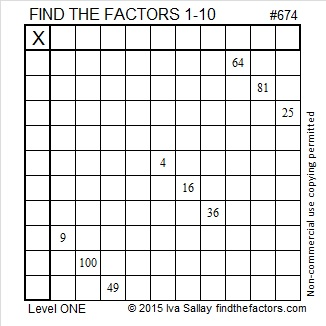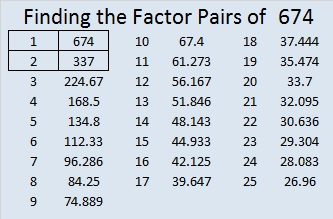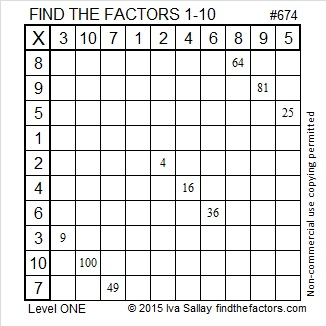# 674 and Level 1

674 is the hypotenuse of the Pythagorean triple 350-576-674. What is the greatest common factor of those three numbers?

674 is a leg in exactly one Pythagorean triple: 674-113568-113570, and those three numbers have the exact same greatest common factor as the triple above.Print the puzzles or type the solution on this excel file: 10 Factors 2015-11-09

—————————————————————————————————

• 674 is a composite number.
• Prime factorization: 674 = 2 x 337
• The exponents in the prime factorization are 1 and 1. Adding one to each and multiplying we get (1 + 1)(1 + 1) = 2 x 2 = 4. Therefore 674 has exactly 4 factors.
• Factors of 674: 1, 2, 337, 674
• Factor pairs: 674 = 1 x 674 or 2 x 337
• 674 has no square factors that allow its square root to be simplified. √674 ≈ 25.96151.—————————————————————————————————This site uses Akismet to reduce spam. Learn how your comment data is processed.